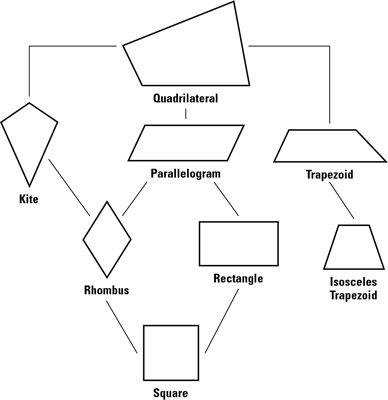##### Geometry Workbook For DummiesA quadrilateral is a polygon with four sides. There are seven quadrilaterals, some that are surely familiar to you, and some that may not be so familiar. Check out the following definitions and the quadrilateral family tree in the following figure.If you know what the quadrilaterals look like, their definitions should make sense and be pretty easy to understand (though the kite definition is a bit of a mouthful). Here are the seven quadrilaterals:

• Kite: A quadrilateral in which two disjoint pairs of consecutive sides are congruent (“disjoint pairs” means that one side can’t be used in both pairs)

• Parallelogram: A quadrilateral that has two pairs of parallel sides

• Rhombus: A quadrilateral with four congruent sides; a rhombus is both a kite and a parallelogram

• Rectangle: A quadrilateral with four right angles; a rectangle is a type of parallelogram

• Square: A quadrilateral with four congruent sides and four right angles; a square is both a rhombus and a rectangle

• Trapezoid: A quadrilateral with exactly one pair of parallel sides (the parallel sides are called bases)

• Isosceles trapezoid: A trapezoid in which the nonparallel sides (the legs) are congruent

In the hierarchy of quadrilaterals shown in the above figure, a quadrilateral below another on the family tree is a special case of the one above it. A rectangle, for example, is a special case of a parallelogram. Thus, you can say that a rectangle is a parallelogram but not that a parallelogram is a rectangle (a parallelogram is only sometimes a rectangle).